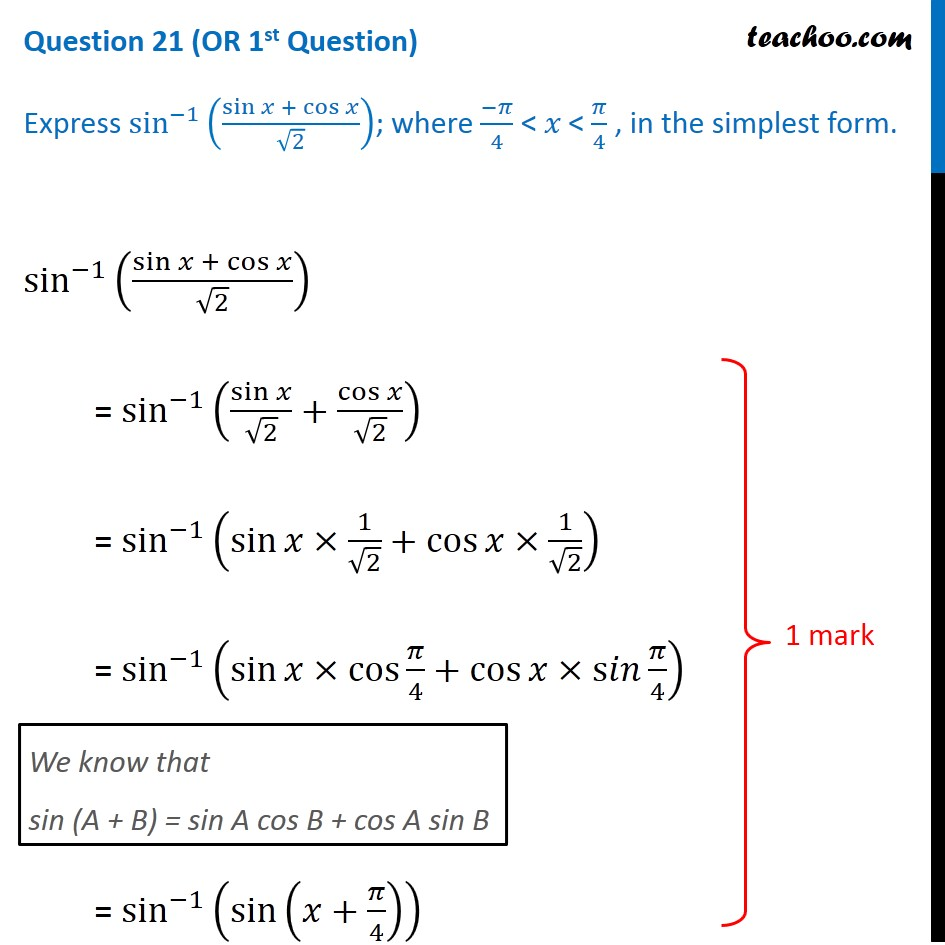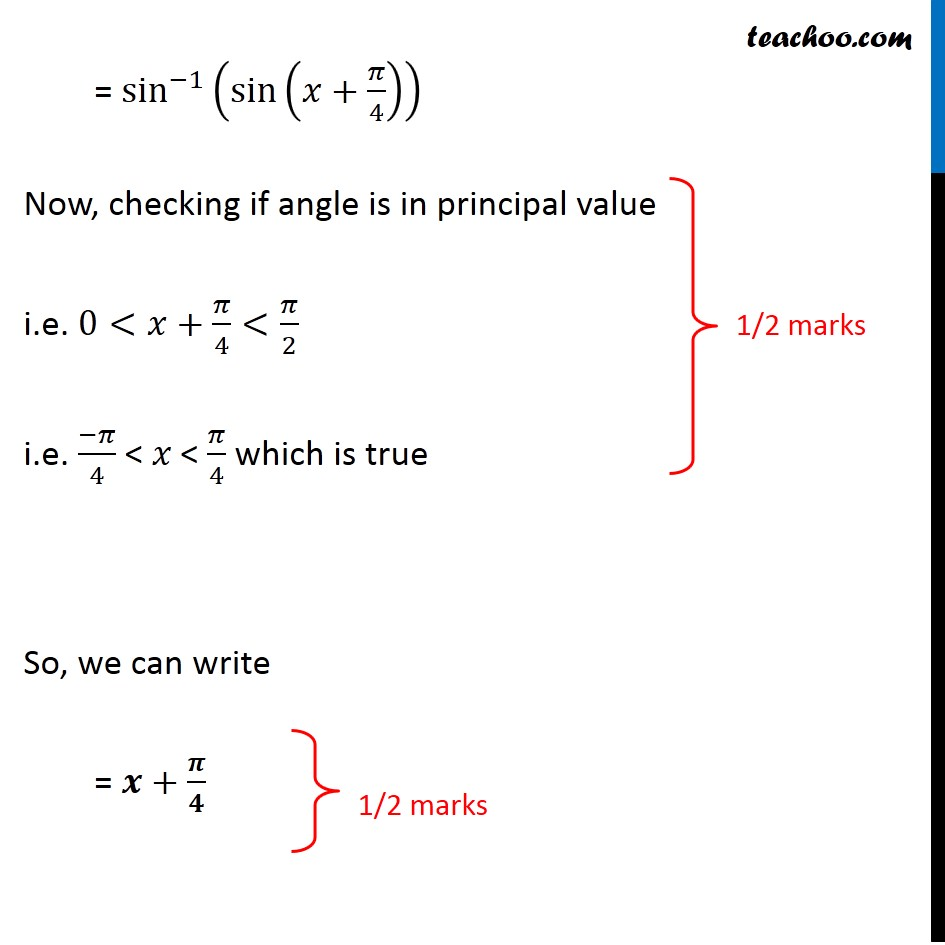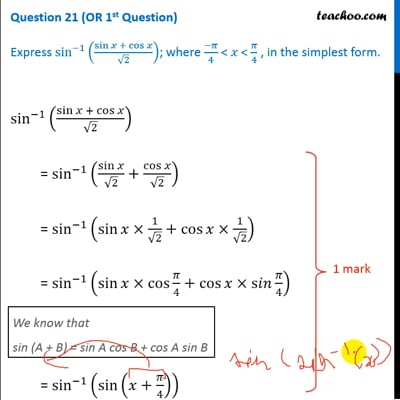CBSE Class 12 Sample Paper for 2020 Boards

Class 12
Solutions of Sample Papers and Past Year Papers - for Class 12 Boards

## Express sin -1 ⁡((sin⁡x  + cos⁡x)/√2); where (-π)/4 < 𝑥 < π/4 , in the simplest form.This video is only available for Teachoo black users

Get live Maths 1-on-1 Classs - Class 6 to 12

### Transcript

Question 21 (OR 1st Question) Express sin^(−1)⁡((sin⁡𝑥 + cos⁡𝑥)/√2); where (−𝜋)/4 < 𝑥 < 𝜋/4 , in the simplest form. sin^(−1)⁡((sin⁡𝑥 + cos⁡𝑥)/√2) = sin^(−1)⁡(sin⁡𝑥/√2+cos⁡𝑥/√2) = sin^(−1)⁡(sin⁡𝑥×1/√2+cos⁡𝑥×1/√2) = sin^(−1)⁡(sin⁡𝑥×cos⁡〖𝜋/4〗+cos⁡𝑥×s𝑖𝑛⁡〖𝜋/4〗 ) We know that sin (A + B) = sin A cos B + cos A sin B = sin^(−1)⁡(sin⁡(𝑥+𝜋/4) ) = sin^(−1)⁡(sin⁡(𝑥+𝜋/4) ) Now, checking if angle is in principal value i.e. 0<𝑥+𝜋/4<𝜋/2 i.e. (−𝜋)/4 < 𝑥 < 𝜋/4 which is true So, we can write = 𝒙+𝝅/𝟒